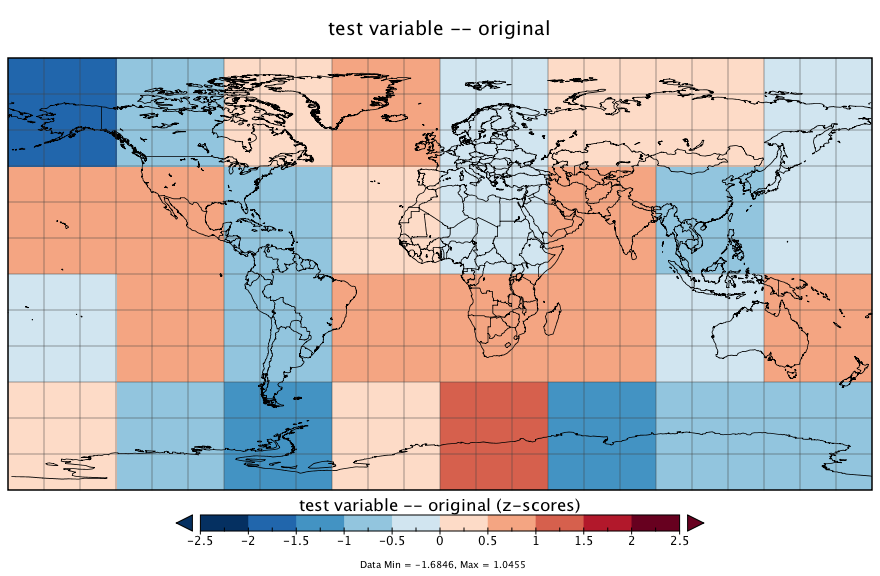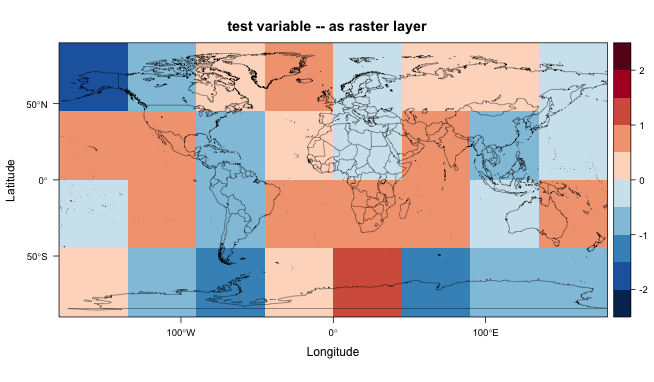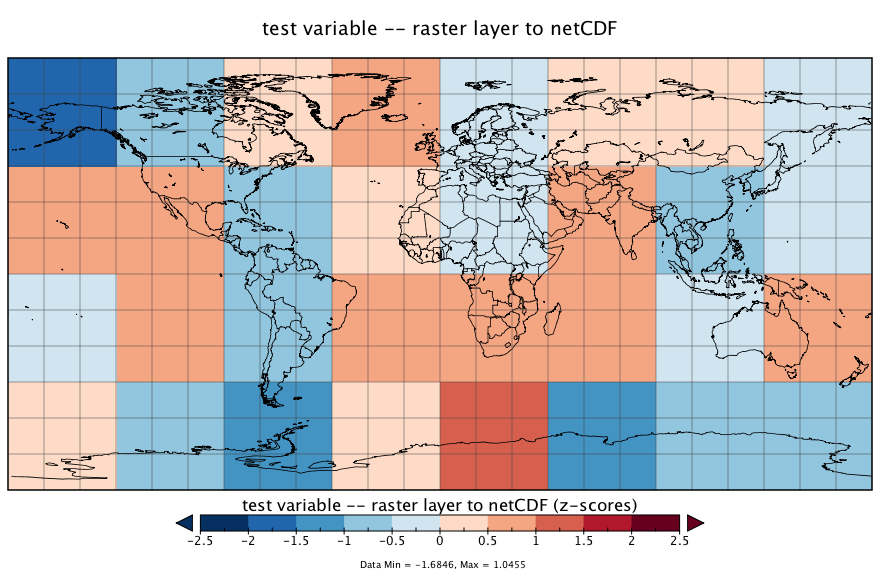NetCDF and the raster package

The raster package has the capability of reading and writing netCDF files. There are several issues that could arise in such transformations (i.e. from the netCDF format to the raster format) related to such things as the indexing of grid-cell locations: netCDF coordinates refer to the center of grid cells, while raster coordinates refer to cell corners.

In practice, the raster package seems to “do the right thing” in reading and writing netCDF, as can be demonstrated using a “toy” data set. In the examples below, a simple netCDF data set will be generated and written out using the ncdf4 package. Then that netCDF data set will be read in as a raster “layer” and plotted, and finally the raster layer will be written again as a netCDF file. As can be seen, the coordinate systems are appropriately adjusted going back and forth between netCDF and the raster “native” format.

Generate and write a simple netCDF file

Generate a small nlon = 8, nlat = 4, 2-d netCDF data set, filled with normally distributed random numbers

library(ncdf4)

# create a small netCDF file, save, and read back in using raster and rasterVis
# generate lons and lats
nlon <- 8; nlat <- 4
dlon <- 360.0/nlon; dlat <- 180.0/nlat
lon <- seq(-180.0+(dlon/2),+180.0-(dlon/2),by=dlon)
lon
##  -157.5 -112.5  -67.5  -22.5   22.5   67.5  112.5  157.5
lat <- seq(-90.0+(dlat/2),+90.0-(dlat/2),by=dlat)
lat
##  -67.5 -22.5  22.5  67.5
# generate some data
set.seed(10) # for reproducibility
tmp <- rnorm(nlon*nlat)
tmat <- array(tmp, dim = c(nlon, nlat))
dim(tmat)
##  8 4
# define dimensions
londim <- ncdim_def("lon", "degrees_east", as.double(lon))
latdim <- ncdim_def("lat", "degrees_north", as.double(lat))

# define variables
varname="tmp"
units="z-scores"
dlname <- "test variable -- original"
fillvalue <- 1e20
tmp.def <- ncvar_def(varname, units, list(londim, latdim), fillvalue,
dlname, prec = "single")

As can be seen, the longitudes run from -157.5 to +157.5, while the latitudes run from -67.5 to +67.5, and they define the centers of the netCDF grid cells.

Write the generated data to a netCDF file.

# create a netCDF file
ncfname <- "test-netCDF-file.nc"
ncout <- nc_create(ncfname, list(tmp.def), force_v4 = TRUE)

# put the array
ncvar_put(ncout, tmp.def, tmat)

# put additional attributes into dimension and data variables
ncatt_put(ncout, "lon", "axis", "X")
ncatt_put(ncout, "lat", "axis", "Y")

title <- "small example netCDF file"
ncatt_put(ncout, 0, "title", title)

# close the file, writing data to disk
nc_close(ncout)

Here’s what the netCDF file looks like, as plotted in Panoply:Read a netCDF file as a raster layer

Now read the netCDF data set back in as a raster object

library(raster)
library(rasterVis)
library(maptools)
library(maps)
# read the netCDF file as a raster layer
tmpin <- raster(ncfname)
tmpin
## class       : RasterLayer
## dimensions  : 4, 8, 32  (nrow, ncol, ncell)
## resolution  : 45, 45  (x, y)
## extent      : -180, 180, -90, 90  (xmin, xmax, ymin, ymax)
## coord. ref. : +proj=longlat +datum=WGS84 +ellps=WGS84 +towgs84=0,0,0
## data source : E:\Dropbox\DataVis\geog495\netcdf-to-raster\test-netCDF-file.nc
## names       : test.variable....original
## zvar        : tmp

Listing the object provides its internal raster attributes, while the print() function provides the characteristics of the source netCDF file.

print(tmpin)
## File E:\Dropbox\DataVis\geog495\netcdf-to-raster\test-netCDF-file.nc (NC_FORMAT_NETCDF4):
##
##      1 variables (excluding dimension variables):
##         float tmp[lon,lat]   (Contiguous storage)
##             units: z-scores
##             _FillValue: 1.00000002004088e+20
##             long_name: test variable -- original
##
##      2 dimensions:
##         lon  Size:8
##             units: degrees_east
##             long_name: lon
##             axis: X
##         lat  Size:4
##             units: degrees_north
##             long_name: lat
##             axis: Y
##
##     1 global attributes:
##         title: small example netCDF file

The data can be mapped using the rasterVis version of the levelplot() function, with continental outlines from the maps package overlain.

# map the data
world.outlines <- map("world", plot=FALSE)
world.outlines.sp <- map2SpatialLines(world.outlines, proj4string = CRS("+proj=longlat"))

mapTheme <- rasterTheme(region = rev(brewer.pal(10, "RdBu")))
cutpts <- c(-2.5, -2.0, -1.5, -1, -0.5, 0, 0.5, 1.0, 1.5, 2.0, 2.5)
plt <- levelplot(tmpin, margin = F, at=cutpts, cuts=11, pretty=TRUE, par.settings = mapTheme,
main="test variable -- as raster layer")
plt + layer(sp.lines(world.outlines.sp, col = "black", lwd = 0.5))Write the raster layer as a netCDF file

Finally, write the raster layer (tmpin) out as a netCDF file:

# write the raster layer (tmpin)
outfile <- "test_raster.nc"
crs(tmpin) <- "+proj=longlat +datum=WGS84 +no_defs +ellps=WGS84 +towgs84=0,0,0"
writeRaster(tmpin, outfile, overwrite=TRUE, format="CDF", varname="tmp", varunit="z-scores",
longname="test variable -- raster layer to netCDF", xname="lon", yname="lat")

Here’s what the resulting netCDF file looks like in Panoply: